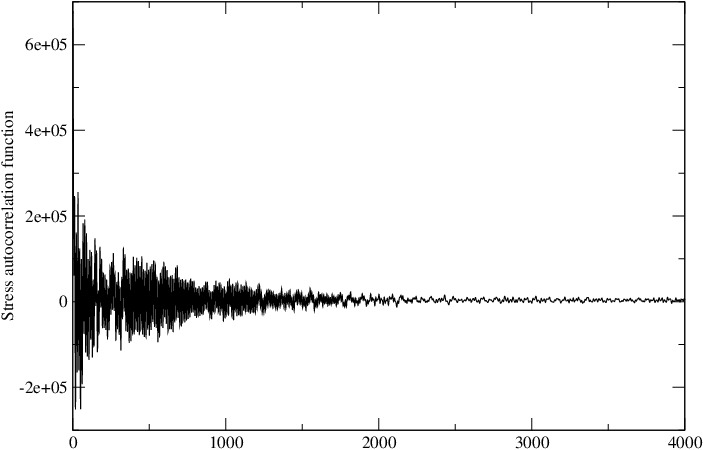Hi,

Perhaps you forgot to send to the mailing list.

If your relaxation time is the parameter characterizing how fast the stress autocorrelation function S(t) decays, you can simply normalize it as S(t)/S(0) and calculate the time integral. Suppose S(t)/S(0) decays as exp(-t/tau), the integral is simply tau, which is a good indicator of the relaxation time.

As for your question regarding the “snapshots”, I am not clear about it. With such long simulation, you should get a very accurate time correlation function because you have about 10^7 time origins for ensemble average. [But your choice of d=ps is not good; I would use d=ps*10.]

With these parameters:
p equal 4000 # correlation length
s equal 1 # sample interval
d equal \$p*\$s # dump interval

You will have 10^7/4000=2500 correlation functions. Do you use “ave one” or “ave running”? I hope you are using the averaged correlation function, not just one of the 2500 correlation functions.

Best,
Bruce

Hello Bruce,

Thank you for your feedback. I forgot to add the mailing list.

By “snapshots”, I meant the number of correlation functions. It is a case of bad terminology, I apologize. In my input file, I used the “ave running”. Now your last statement sheds more light on my second question. The image I showed is the last of the 2500 correlation functions. Now are you saying, using this last function will be inaccurate? And will need to average over all the 2500?

Thank you once again.Hi,

Using “ave running” means that the last correlation function is the final averaged one. So it’s good to use the last one.

Best,
Bruce

Thank you very much, Bruce. I really appreciate this.